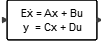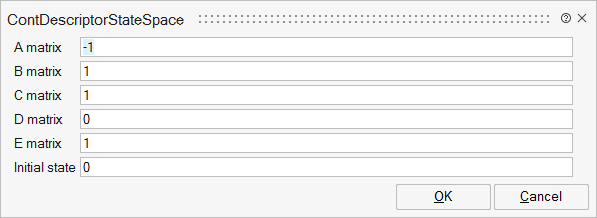# ContDescriptorStateSpace

This block implements a continuous-time, linear, implicit state-space system specified by the A, B, C, D, E matrices, the initial state vector, and the equation, E*der(x)=A*x+B*u.## Description

This block implements a continuous-time implicit state-space system govorned by following equations:## ParametersNameLabelDescriptionData TypeValid Values

A

A matrix

Real nxn matrix where n is the number of states, default value -1.

Matrix

B

B matrix

Real nxm matrix where n is the number of states and m is the number of inputs, default value: 1. B=[ ] if block has no input.

Matrix

C

C matrix

Real rxn matrix, default value: 1. r is the number of outputs and n is the number of states.

Matrix

D

D matrix

Real rxm matrix where r is the number of outputs and m is the number of inputs, default value: 0.

Matrix

E

E matrix

Real nxn matrix where n is the number of states, default value 1. This matrix can be singular.

Matrix

x0

Initial state

Vector of size n or scalar (expanded to a vector of size n).

Matrix

## Ports

NameTypeDescriptionIO TypeNumber

Port 1

explicit

output

1

Port 2

explicit

input

1

NameValueDescription

always active

yes

direct-feedthrough

no, except when D not zero

zero-crossing

no

mode

no

continuous-time state

yes, except when n is zero

discrete-time state

no